Refer to our Texas Go Math Grade 1 Answer Key Pdf to score good marks in the exams. Test yourself by practicing the problems from Texas Go Math Grade 1 Lesson 1.3 Answer Key Tens.

Essential Question
How can you model and name groups of ten?
Explanation:
By using ten frame and base ten Blocks ones.

Explore
Use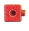to solve the riddle.
Draw and write to show your work.

For The Teacher

• Read the following riddles. I am thinking of a number that is the same as 1 ten and 4 ones. What is my number? I am thinking of a number that is the same as 1 ten and 0 ones. What is my number?
First number is 14
Second number is 10
1)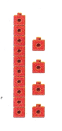2)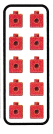Math Talk
Mathematical processes
Explain what you did to solve the first riddle.
Explanation:
First riddle is 1 ten that is 10 ones
and 4 ones
10 + 4 = 14

Model and Draw

Draw a quick picture to show the tens.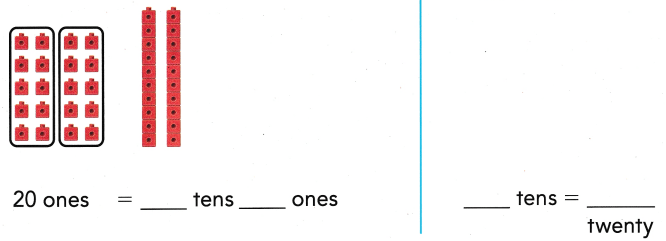2 tens = 20 ones
Explanation:
Converting tens to ones
by Base ten blocks method

Share and Show

Use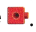Make groups of ten. Draw the tens. Write the tens and ones.

Draw the tens.
Count by tens.

Question 1.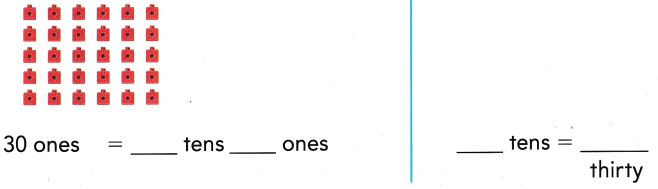Explanation:
Converting ones to tens
by Base ten blocks method
3 tens = 30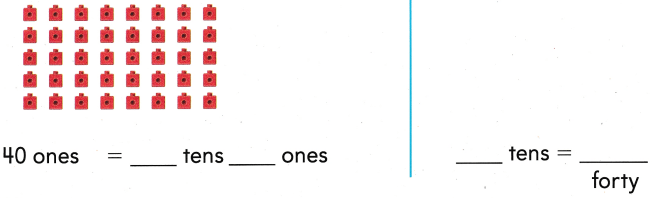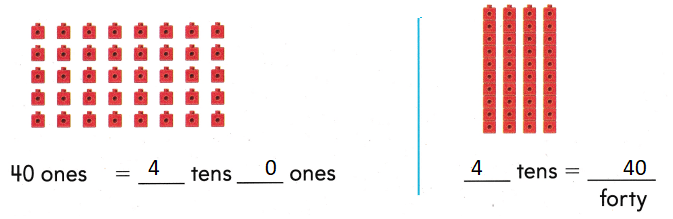Explanation:
Converting ones to tens
by Base ten blocks method
4 tens = 40

Problem Solving

Use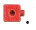Make groups of ten. Write the tens and ones.

Draw the tens. Count by tens.

Question 3.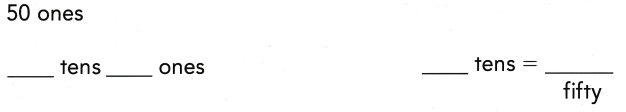Explanation:
Converting ones to tens
by Base ten blocks method
5 tens = 50

Question 4.Explanation:
Converting ones to tens
by Base ten blocks method
6 tens = 60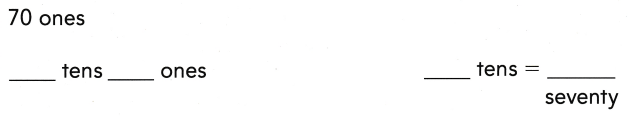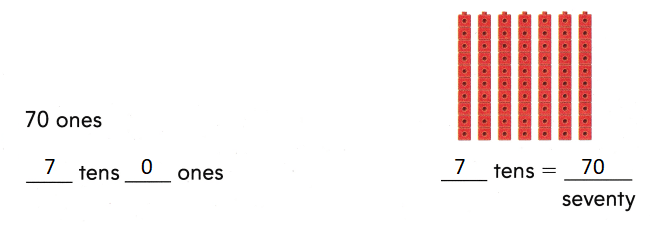Explanation:
Converting ones to tens
by Base ten blocks method
7 tens = 70

Draw a quick picture to show the number. Write the numbers.

Question 6.
H.O.T. Tyler has blue, red, yellow, and purple beads. He has 10 beads of each color. How many beads does Tyler have?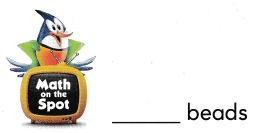Explanation:
3 tens = 30
Tyler have is 30

Question 7.
H.O.T Multi-Step Eli has 3 tens. Nora has 2 tens. Then Sally put 5 tens with the tens. What number did they make?
______ tens = ____
10 tens = 100 (Hundred)
Explanation:
Eli has 3 tens. Nora has 2 tens. Then Sally put 5 tens with the tens.
3 tens = 30
2 tens = 20
5 tens = 50
30 + 20 + 50 = 100
10 tens

Question 8.
Use Tools Chris rolls a bowling ball. The ball hits 10 bowling pins. Chris scores 30 points.
Which model shows 30?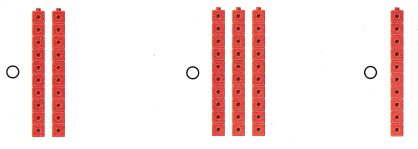Explanation:
Chris scores 30 points.
3 tens = 30

Question 9.
Multi-Step The green team has 20 points. The purple team has 20 points. Then the green team scored 10 more points. Which shows the total points scored?
(A) 10
(B) 60
(C) 50
The green team has 20 points. The purple team has 20 points.
20 + 20 = 40
40 +10 = 50 points scored

Texas Test Prep What number does the model show?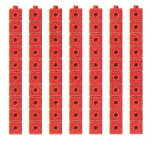(A) 60
(B) 7
(C) 70
Explanation:
70 is the number that model show.
7 tens = 70

Take Home Activity

• Have your child count out small objects into groups of ten, tell how many tens in all, and then say the number word.
Explanation: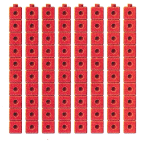Eighty

### Texas Go Math Grade 1 Lesson 1.3 Homework and Practice Answer Key

Use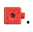Make groups of ten. Write the tens and ones.

Question 1.Explanation:
Converting ones to tens
by Base ten blocks method
6 tens and 0 ones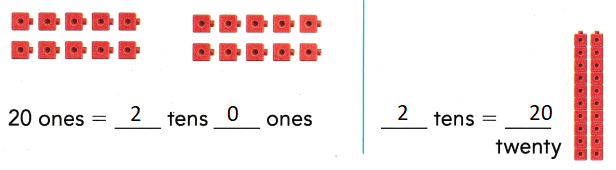Explanation:
Converting ones to tens
by Base ten blocks method
2 tens and 0 ones

Problem Solving

Question 3.
Jim has 5 colors of shells. He has 10 shells of each color. How many shells does Jim have?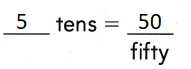Explanation:
5 tens = 50
50 shells that Jim have

Texas test Prep

Lesson Check

Question 4.
Mio had ten bags. The bags held 40 marbles. Which model shows 40?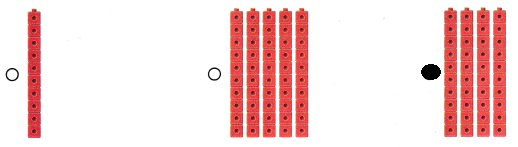Explanation:
4 tens = 40
Bubbled the correct option.

What number does the model show?(A) 80
(B) 10
(C) 8
Explanation:
8 tens = 80
Number 10 the model show

Question 6.
Chen lined up blocks to show a number. What is the number?(A) 10
(B) 30
(C) 3
Explanation:
Chen lined up blocks to show a number.
the number is 30

Texas Go Math Answer Key Grade 1 Lesson 1.3 Homework Question 17.
Multi-Step A box of crayons has ten crayons. Jan has 4 boxes. Phil has 2 boxes. Liz has 3 boxes. Draw a quick picture to show the number. How many crayons do they have?
(A) 9
(B) 60
(C) 90